#2018 iT 邦幫忙鐵人賽DAY 1
5
Modern Web

# 0. 今日工事

• 吉祥話
• Particle System
• 探索流星源代碼 Trace Code
• 宇宙是顆球的話
• 神秘銀河系# 1. 吉祥話# 3. 環境建立

Yo！我找到了！

``````three-stars/
├── 01
│   ├── index.html
│   ├── js
│   │   ├── GPUParticleSystem.js
│   │   ├── controls
│   │   │   └── TrackballControls.js
│   │   └── libs
│   │       ├── dat.gui.min.js
│   │       └── stats.min.js
│   └── textures
│       ├── particle2.png
│       └── perlin-512.png
└── build
└── three.js

6 directories, 8 files
``````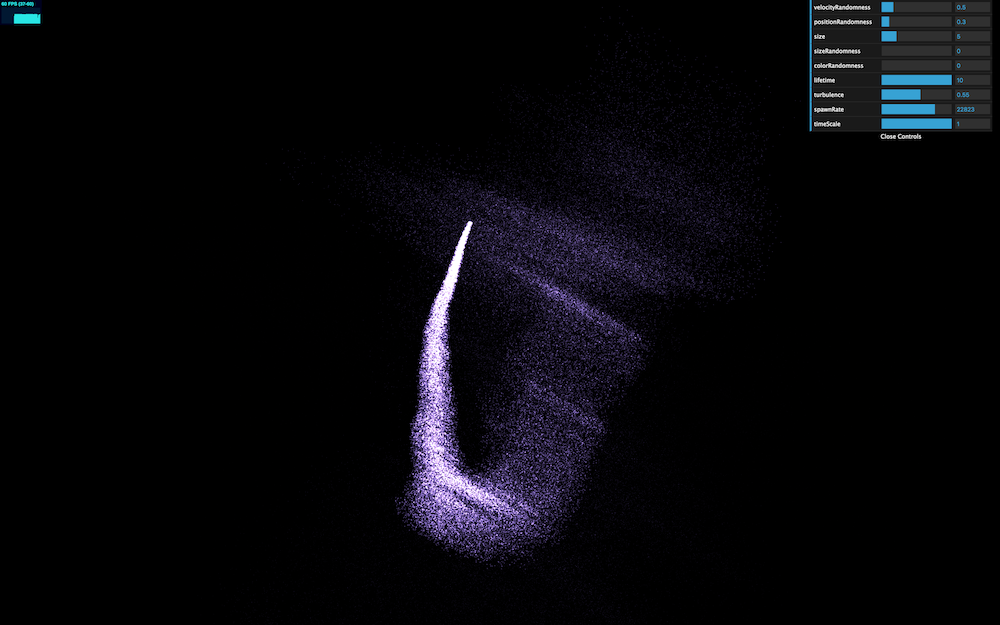# 4. 探索流星源代碼 Trace Code

`index.html`裡面，前面落落長其實只不過是設定一堆東西，相信只要耐心閱讀 doc 就可以理解。實際源代碼的執行就是下面這兩個函式，`init`設定環境，而`animate`裡面則進行`requestAnimationFrame( animate );`讓畫面不斷進行。

``````init();
animate();
``````

• 設定位置:
``````options.position.x = Math.sin( tick * spawnerOptions.horizontalSpeed ) * 20;
options.position.y = Math.sin( tick * spawnerOptions.verticalSpeed ) * 10;
options.position.z = Math.sin( tick * spawnerOptions.horizontalSpeed + spawnerOptions.verticalSpeed ) * 5;
``````
• 產生 particle:
``````for ( var x = 0; x < spawnerOptions.spawnRate * delta; x++ ) {
particleSystem.spawnParticle( options );
}
``````

# 5. 宇宙是顆球的話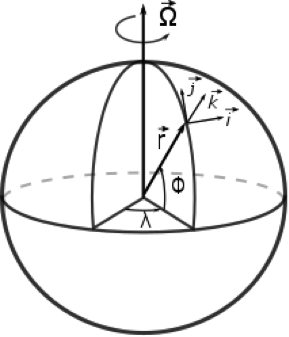``````function sphere() {
const r = 40;
const theta = Math.random() * Math.PI * 2;
const phi = Math.random() * Math.PI;
const xpos = r * Math.sin(phi) * Math.cos(theta);
const ypos = r * Math.sin(phi) * Math.sin(theta);
const zpos = r * Math.cos(phi);

return ({
x: xpos,
y: ypos,
z: zpos,
});
}
``````

``````for ( var x = 0; x < spawnerOptions.spawnRate * delta; x++ ) {
options.position = sphere();
particleSystem.spawnParticle( options );
}
``````

• 原版：
``````options = {
position: new THREE.Vector3(),
positionRandomness: .3,
velocity: new THREE.Vector3(),
velocityRandomness: .5,
color: 0xaa88ff,
colorRandomness: .2,
turbulence: .5,
size: 5,
sizeRandomness: 1
};

spawnerOptions = {
spawnRate: 15000,
horizontalSpeed: 1.5,
verticalSpeed: 1.33,
timeScale: 1
};
``````
• 調整後：
``````options = {
position: new THREE.Vector3(),
positionRandomness: .3,
velocity: new THREE.Vector3(),
velocityRandomness: .5,
color: 0xaa88ff,
colorRandomness: .2,
turbulence: 1.0,
size: 5,
sizeRandomness: 1
};

spawnerOptions = {
spawnRate: 30000,
horizontalSpeed: 10,
verticalSpeed: 10,
timeScale: 0.1,
};
``````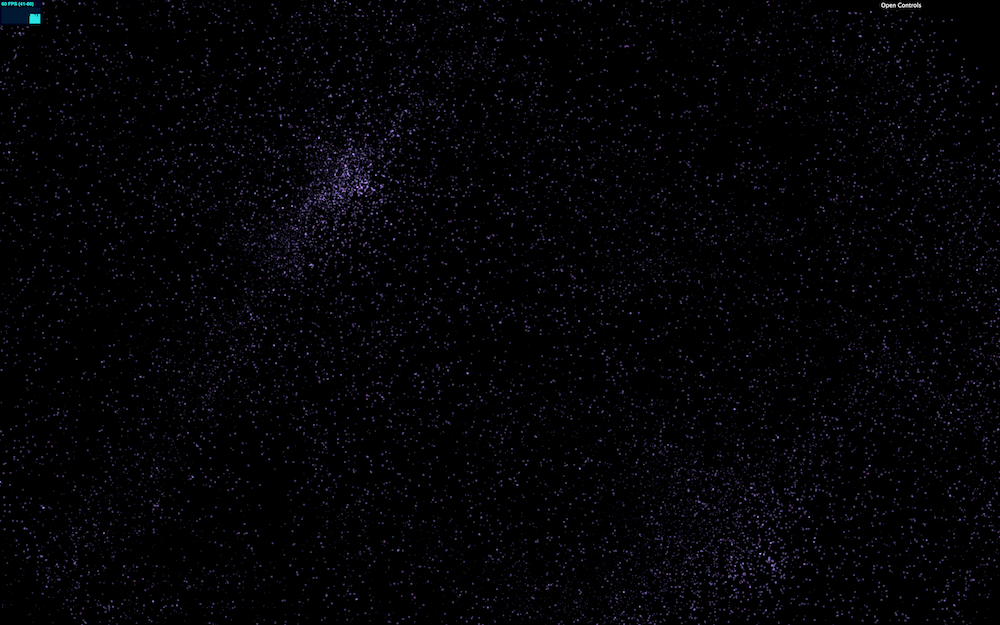# 6. 神秘銀河系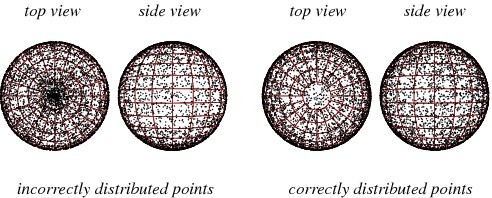``````function sphereEvenly() {
const r = 40;
const theta = Math.random() * Math.PI * 2;
const zpos = Math.random() * 2 - 1;
const rsin = Math.sqrt(1 - zpos * zpos)
const xpos = rsin * Math.cos(theta);
const ypos = rsin * Math.sin(theta);

return ({
x: r * xpos,
y: r * ypos,
z: r * zpos,
});
}
``````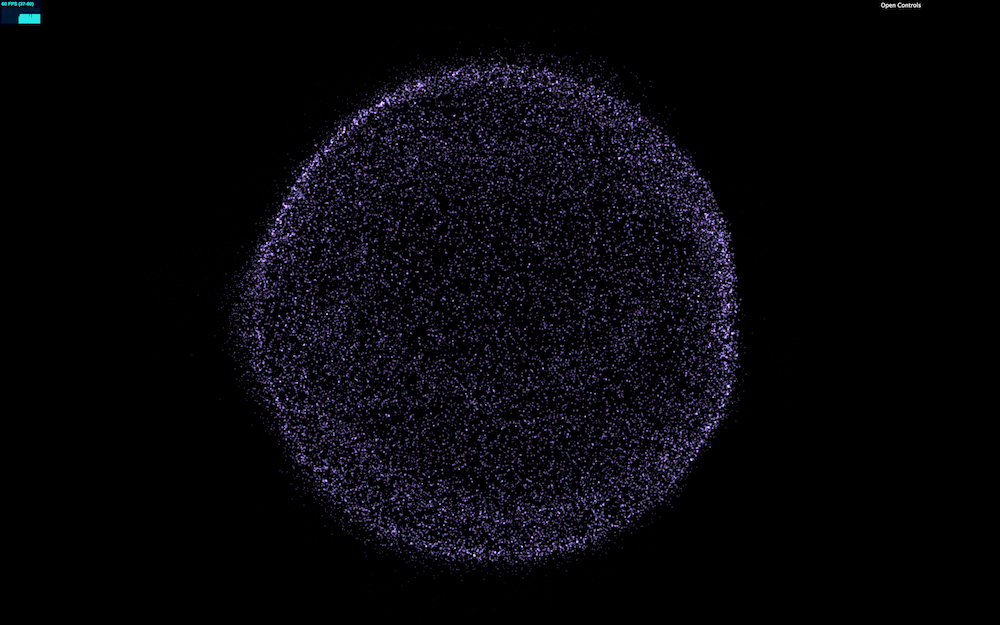# 7. 請愛ＣＹＢＥＲの ａｕｄｉｏ / ＶＩＳＵＡＬ

## Vibert Thio

§ｄ２§ 錐細胞衝擊！時空扭曲！數學模擬黑洞造型。